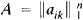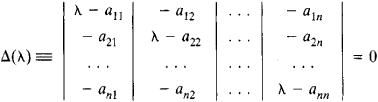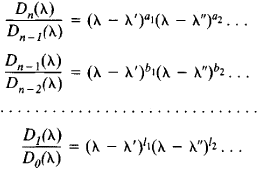# Elementary Divisor

(redirected from Smith normal form)
Also found in: Wikipedia.
The following article is from The Great Soviet Encyclopedia (1979). It might be outdated or ideologically biased.

## Elementary Divisor

The elementary divisors of a square matrixare the polynomials (λ – λ1)p1, (λ – λ2)p2, . . ., (λ – λs)ps obtained from the characteristic equationThe minors of the kth-order determinant Δ(λ), kn, are polynomials in λ. Let Dk (λ), k = 1, 2,. . ., n, be the greatest common divisor of these polynomials, with Dn (λ) ≡ Δ(λ). In the sequence

D0(λ) ≡ 1, D1(λ), D2(λ),...,Dn(λ)

each polynomial is divisible by the preceding one without remainder. Let the corresponding quotients be expressed as a product of linear factors in the field of complex numbers:The polynomials (λ – λ′)a1, (λ – λ″)a2, . . ., (λ – λ′)l1, (λ – λ″)l2, . . . form the complete system of elementary divisors of A (here, powers with zero exponents are not taken into consideration).

The product of all elementary divisors is equal to the characteristic polynomial. The elementary divisors determine the Jordan form of A.